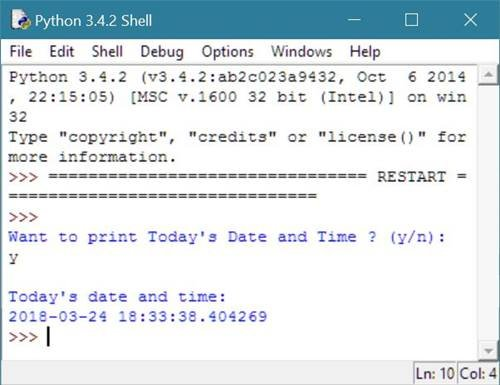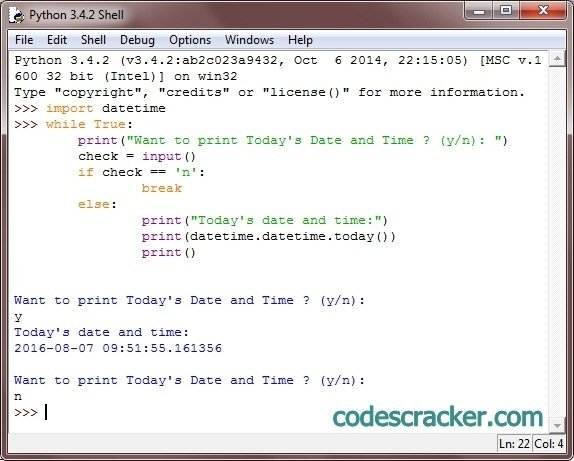# Python Program to Print Date and Time

## Print Date and Time in Python

To print date and time in python, you have to first import the datetime library and use datetime.datetime.today() code inside the print() statement to print the today's current date and time as output as shown here.

## Python Programming Code to Print Date and Time

Following python program prints the current date and time:

```# Python Program - Print Date and Time

import datetime;
print("Want to print Today's Date and Time ? (y/n): ");
check = input();
if check == 'n':
exit();
else:
print("\nToday's date and time:");
print(datetime.datetime.today());
```

Here is the sample run of the above python program to illustrate how to print current date and time:Now let's supply y as input and press enter key to see the current date and time:Same program on python shell:### Same Program in Other Languages

You may also like to learn or practice the same program in other popular programming languages:

Tools
Calculator